# Selina Solutions Concise Maths Class 10 Chapter 16 Loci (Locus and its Constructions)

Locus is defined as the path traced by a moving point which satisfies a certain given condition/conditions. Theorems based on symmetry, applications and its constructions are studied in this Chapter. For students wanting an after-school reference for solving the exercise problems, the Selina Solutions for Class 10 Mathematics is the best resource a student can get. All the solutions are created by subject experts at BYJU’S, keeping in mind the latest ICSE marking patterns. The Selina Solutions Concise Maths Class 10 Chapter 16 Loci (Locus and its Constructions) PDF can be downloaded easily from the link below.

## Selina Solutions Concise Maths Class 10 Chapter 16 Loci (Locus and its Constructions) Download PDF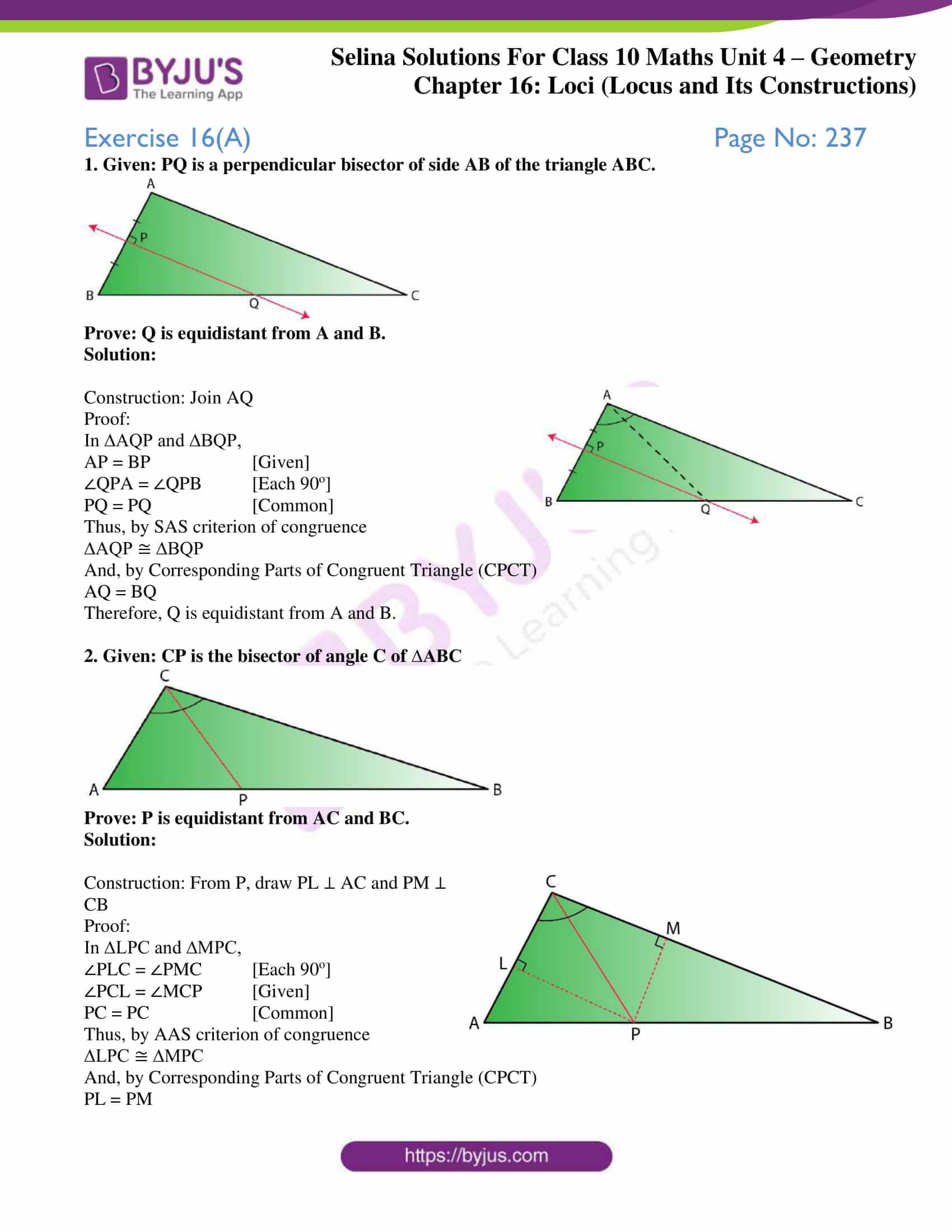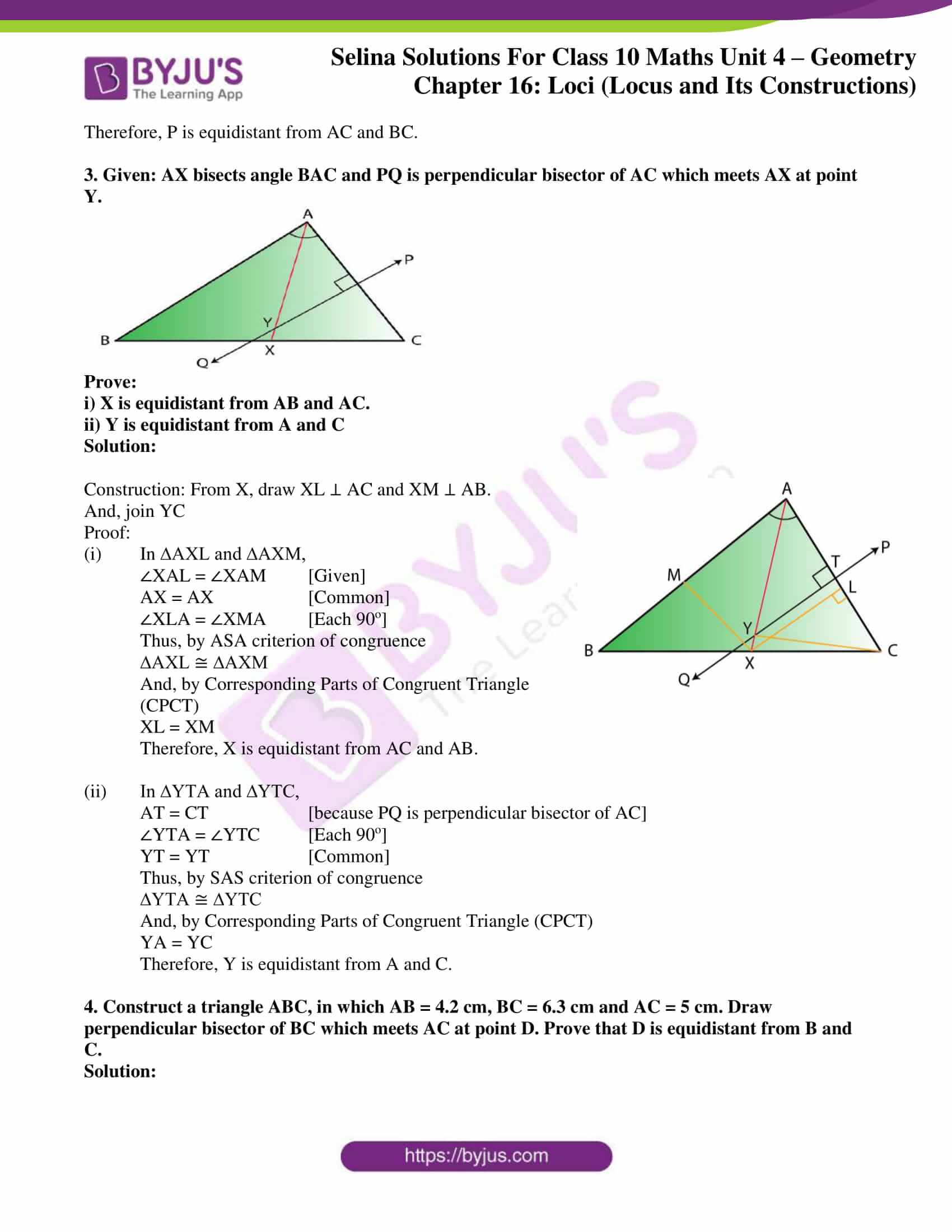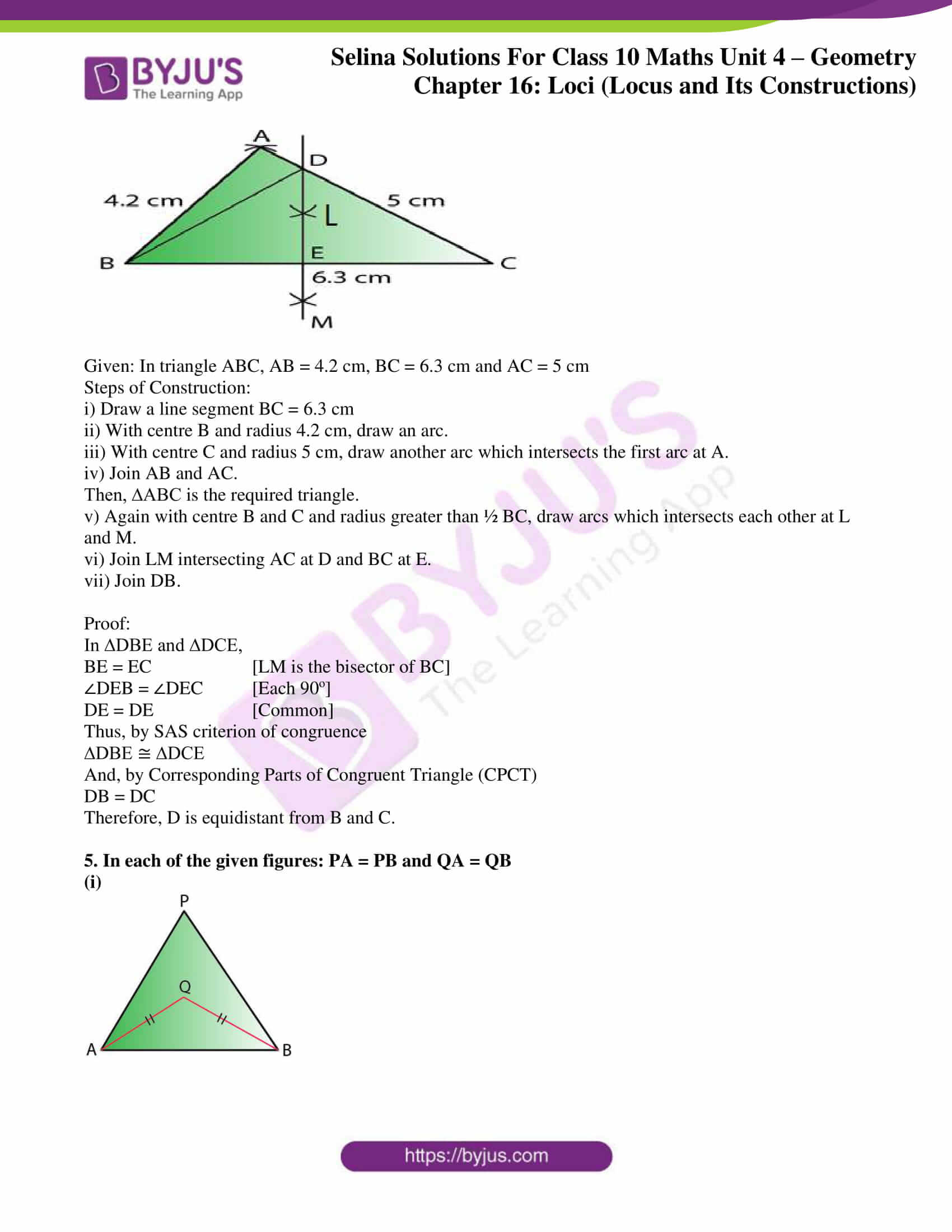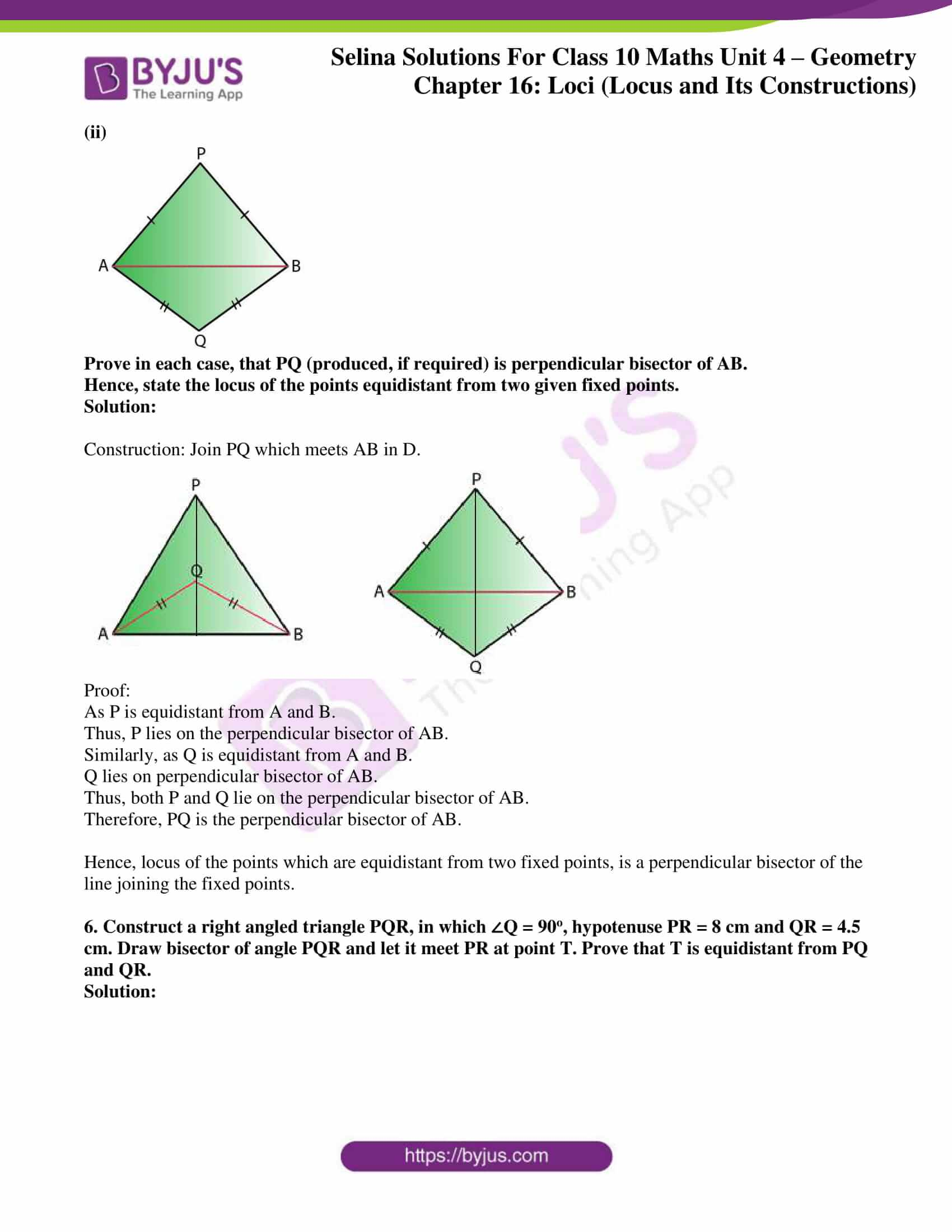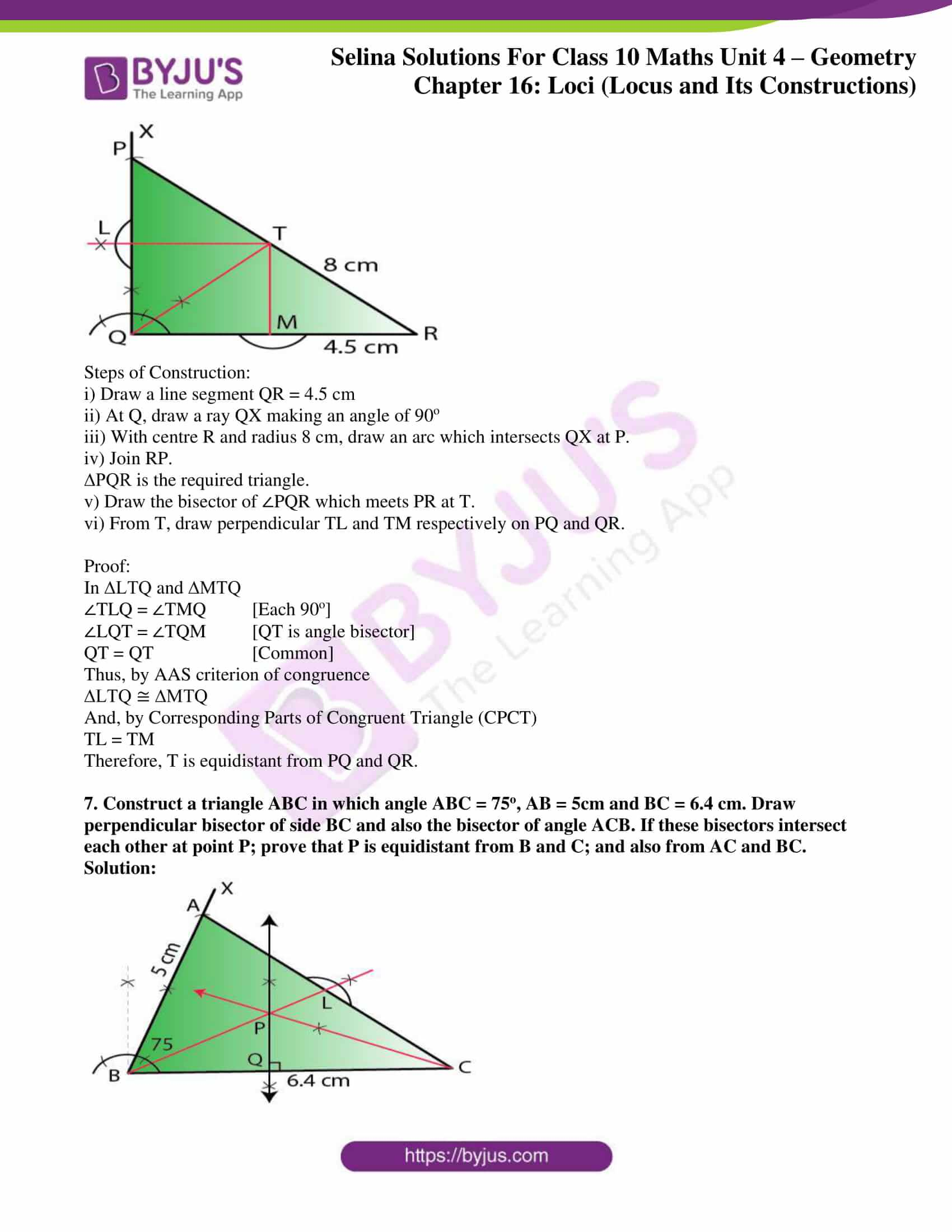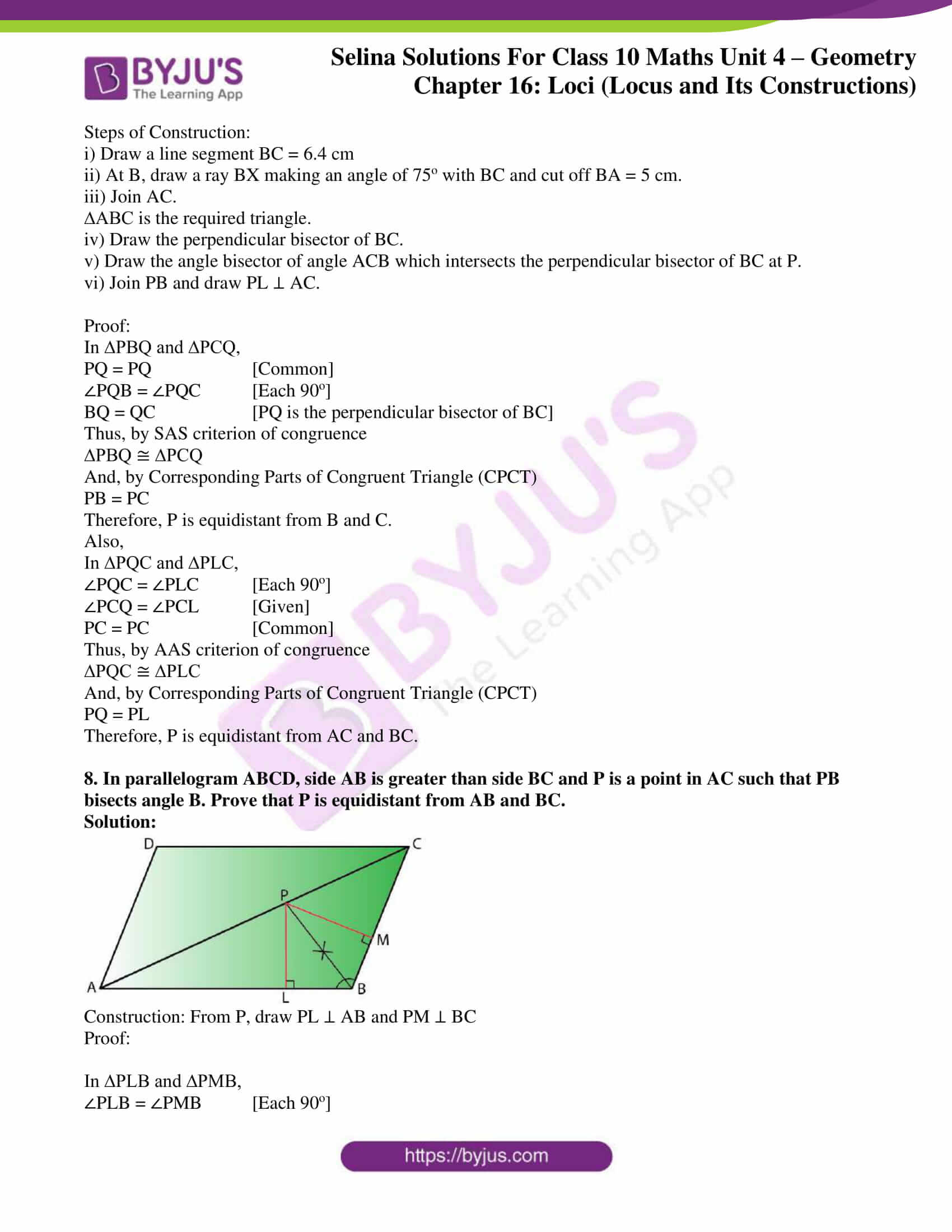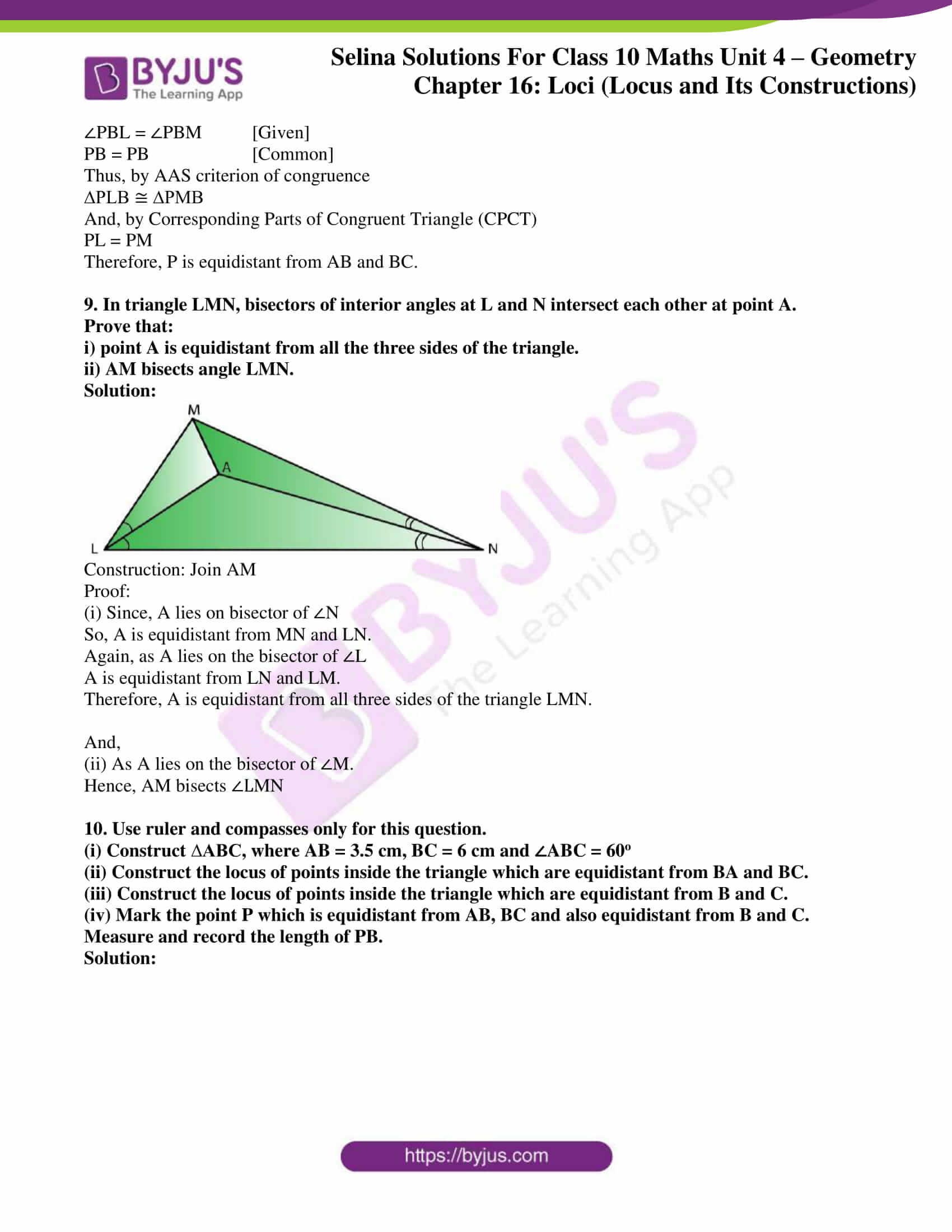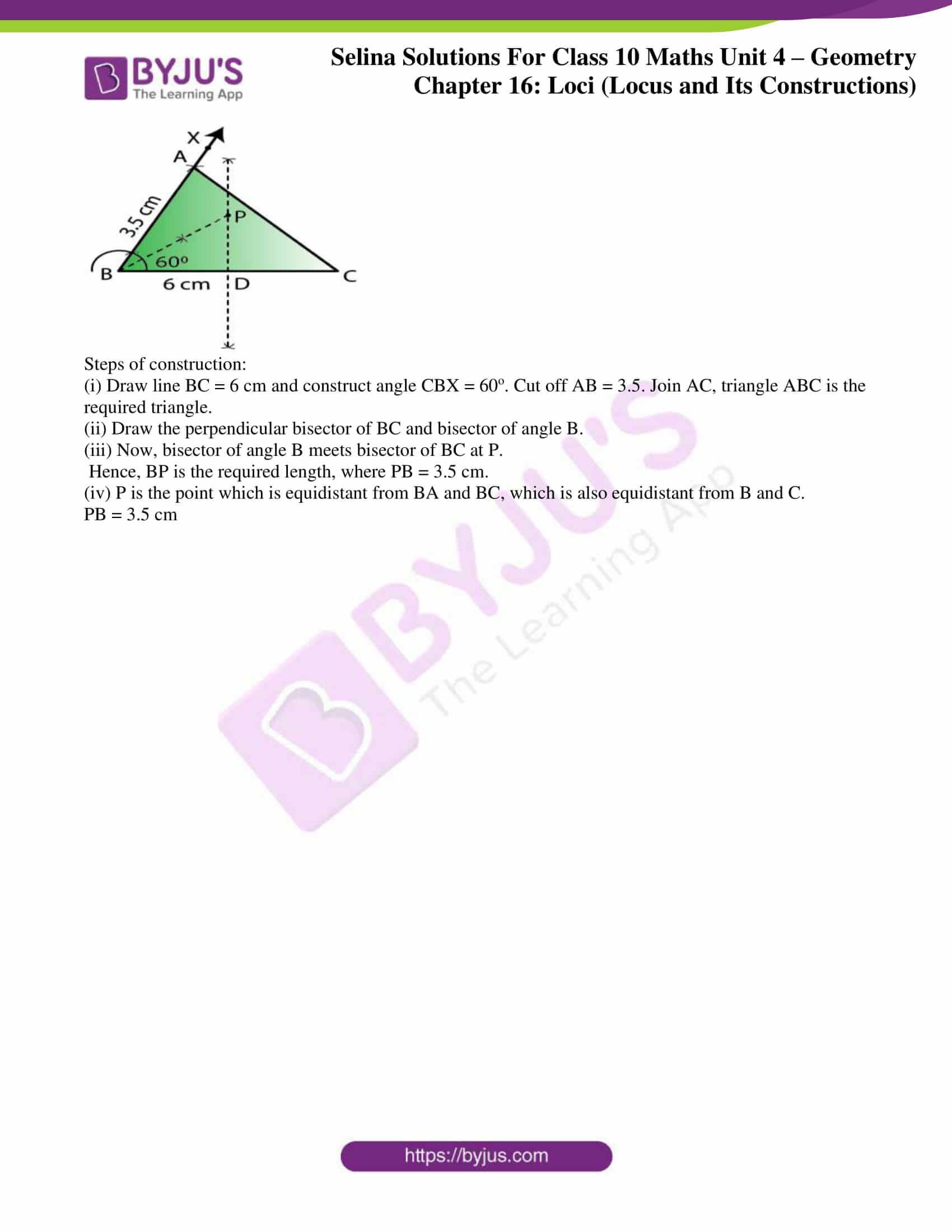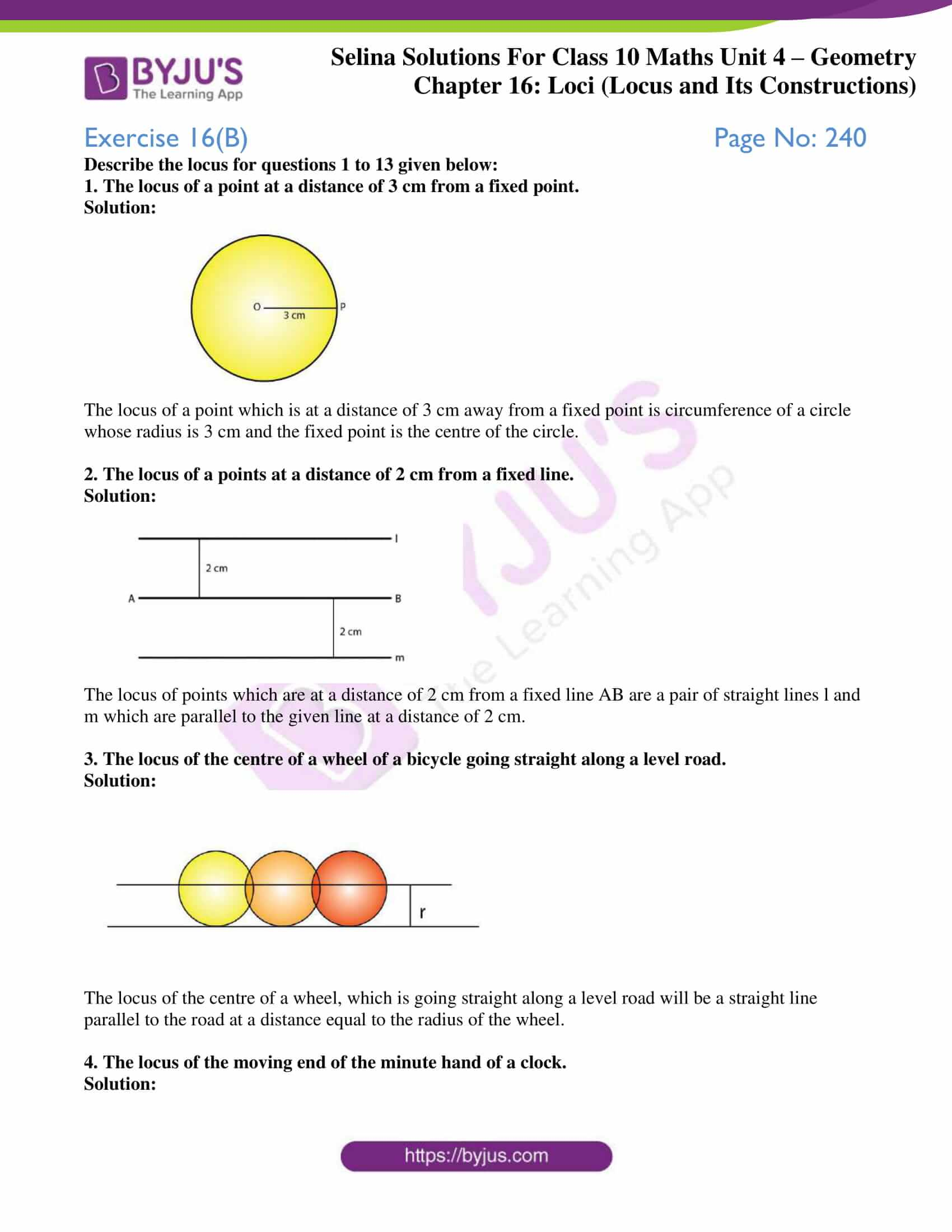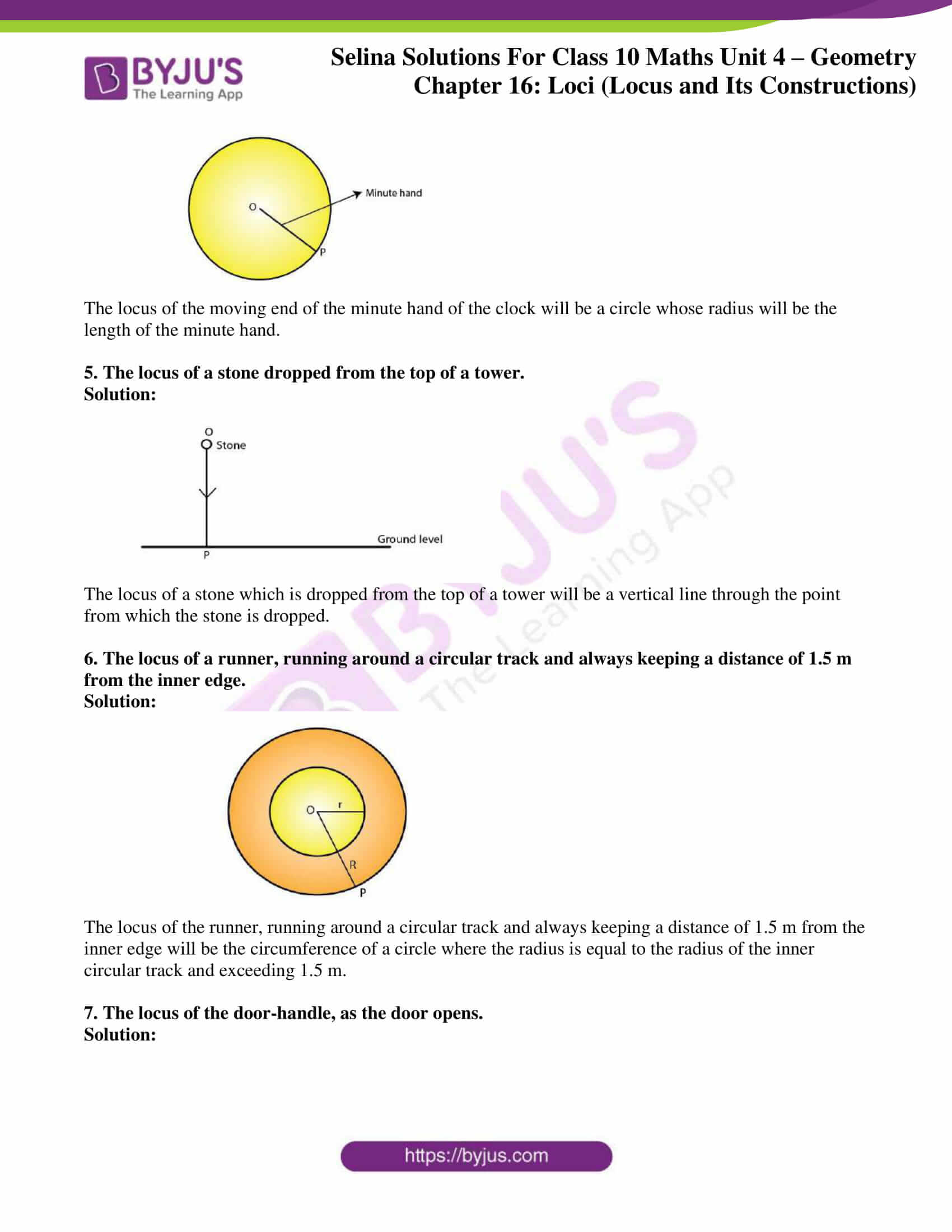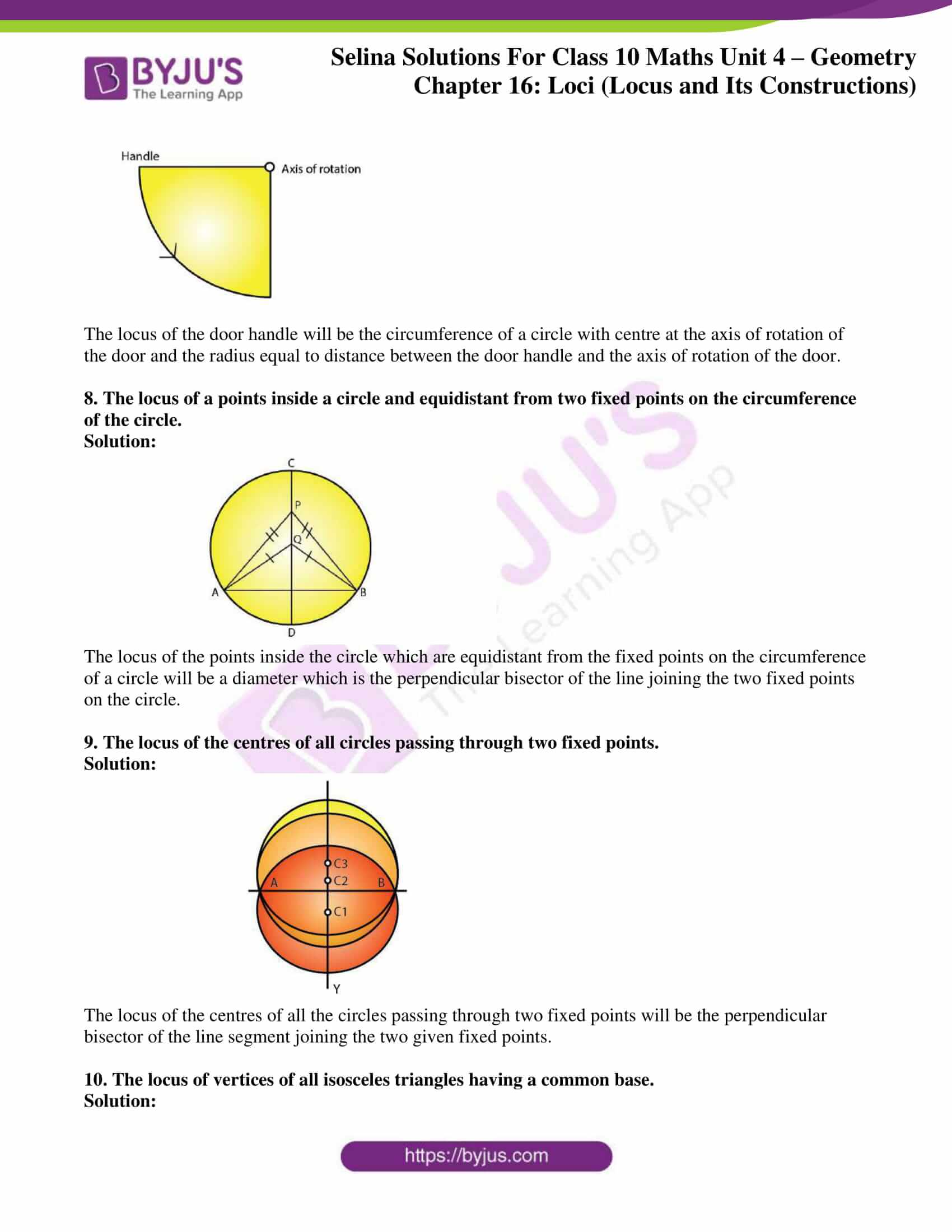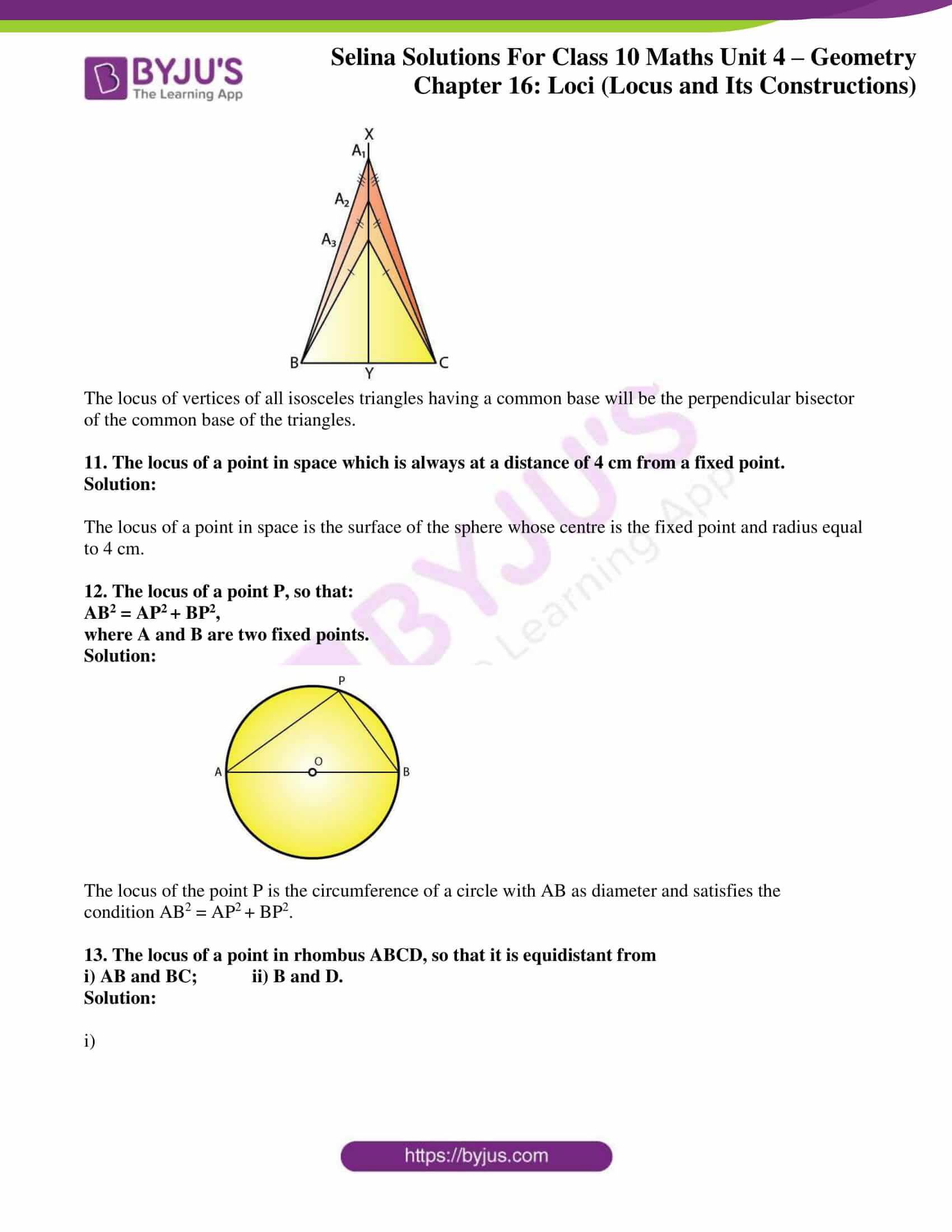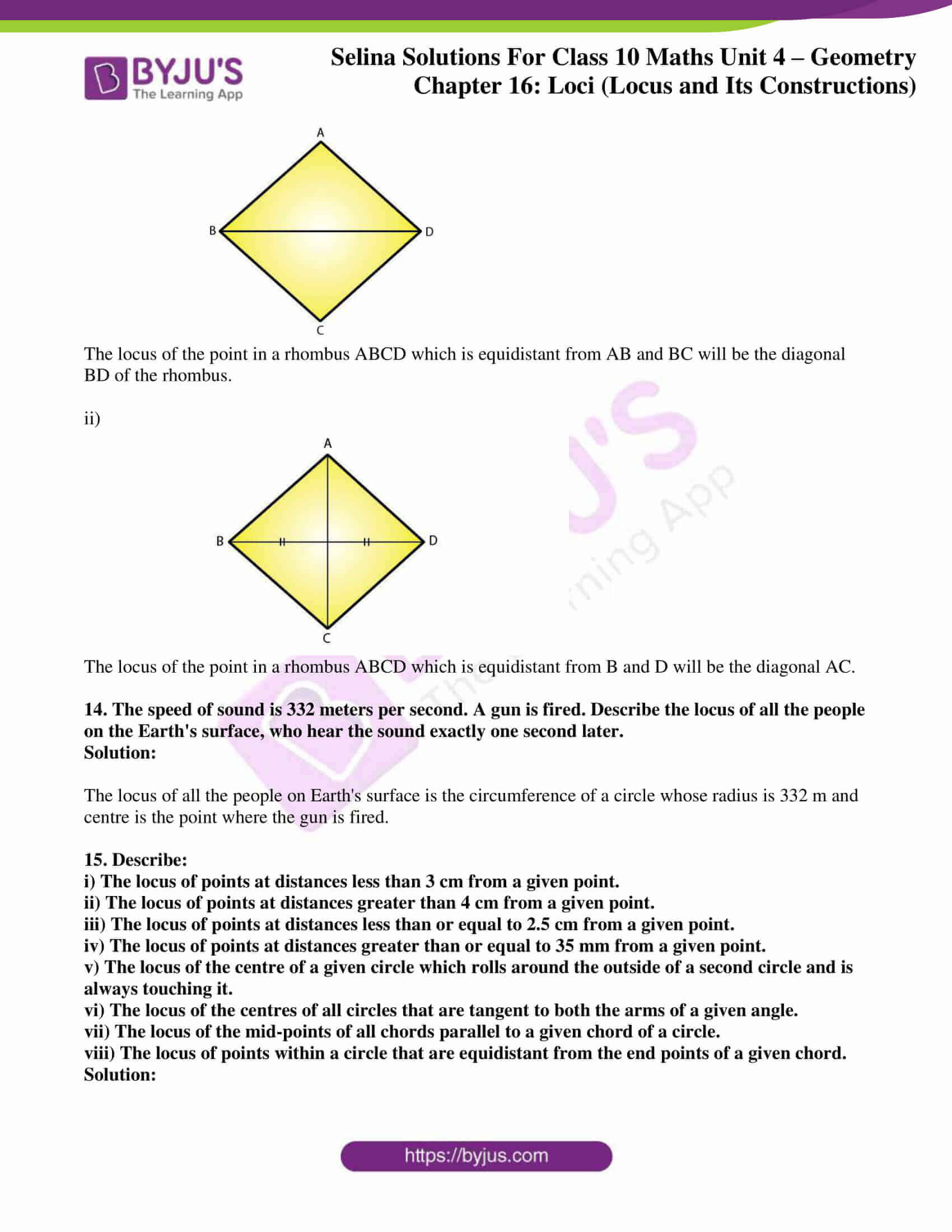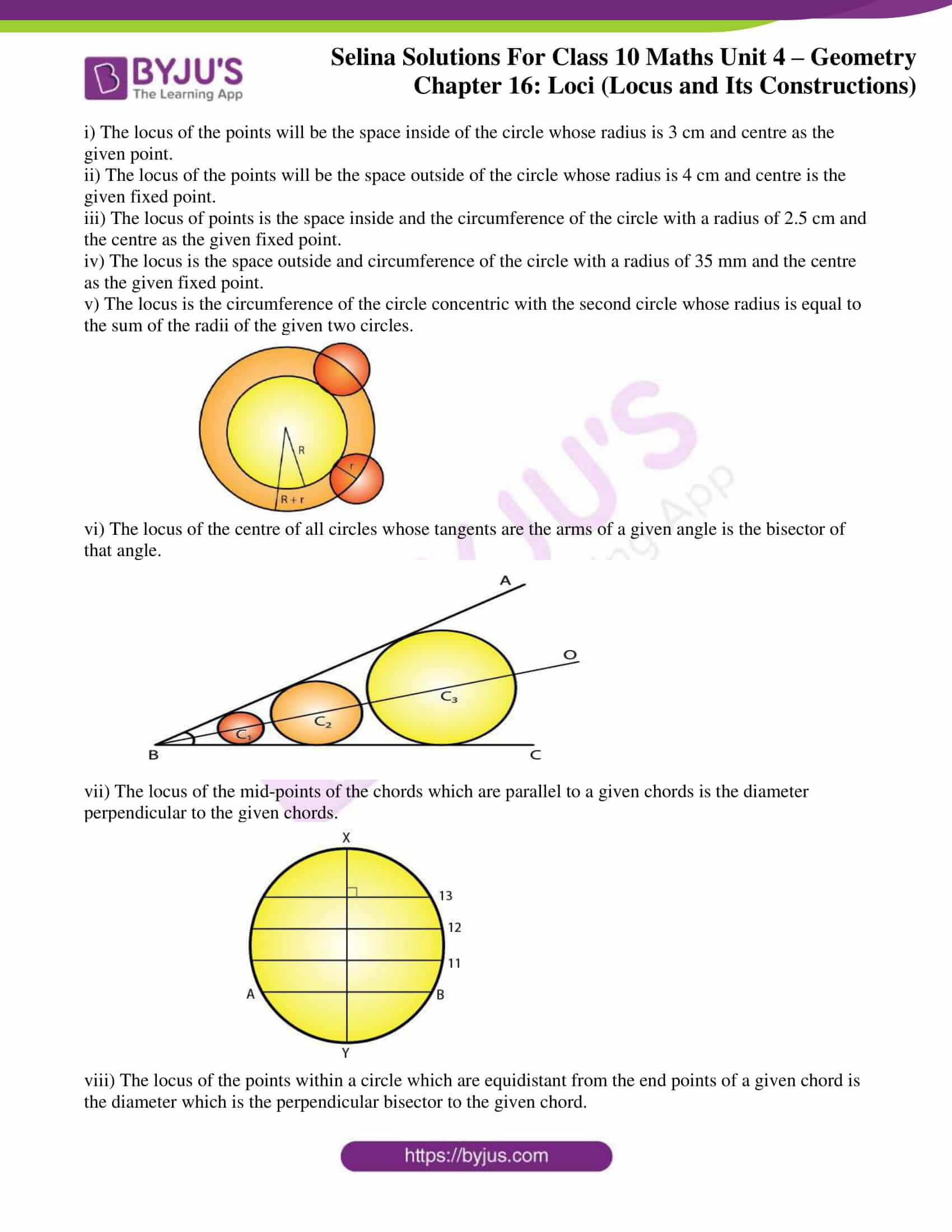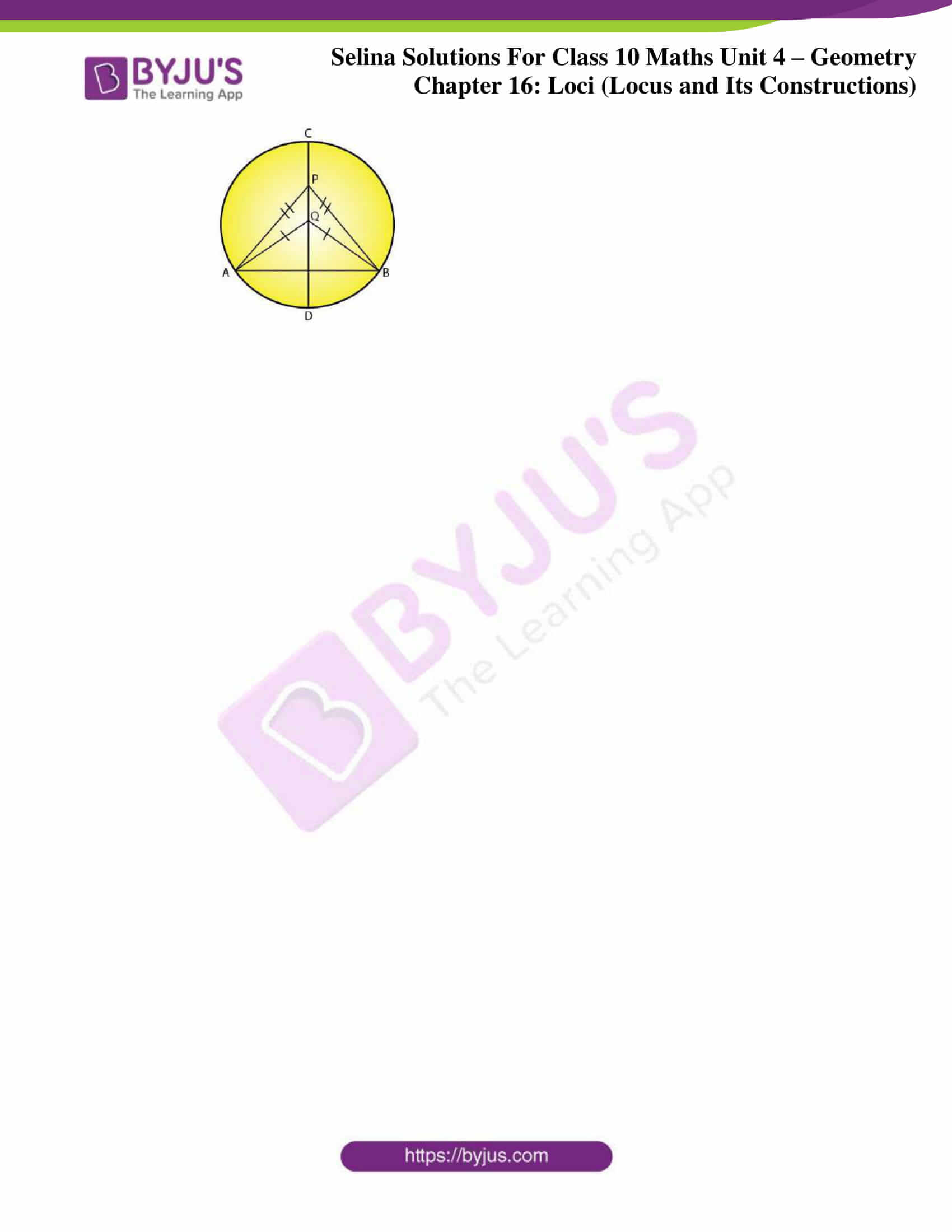## Access Selina Solutions Concise Maths Class 10 Chapter 16 Loci (Locus and its Constructions)

#### Exercise 16(A) Page No: 237

1. Given: PQ is a perpendicular bisector of side AB of the triangle ABC.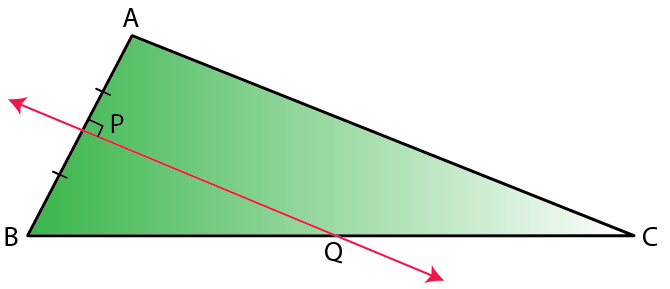Prove: Q is equidistant from A and B.

Solution: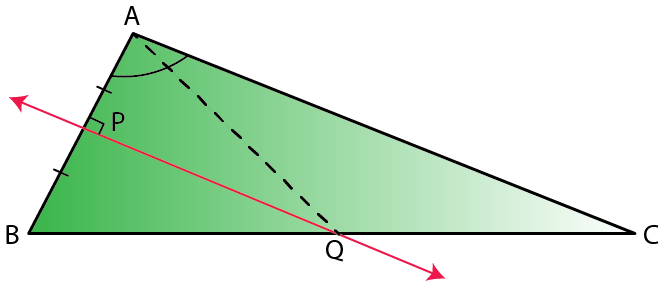Construction: Join AQ

Proof:

In ∆AQP and ∆BQP,

AP = BP [Given]

∠QPA = ∠QPB [Each 90o]

PQ = PQ [Common]

Thus, by SAS criterion of congruence

∆AQP ≅ ∆BQP

And, by Corresponding Parts of Congruent Triangle (CPCT)

AQ = BQ

Therefore, Q is equidistant from A and B.

2. Given: CP is the bisector of angle C of ∆ABC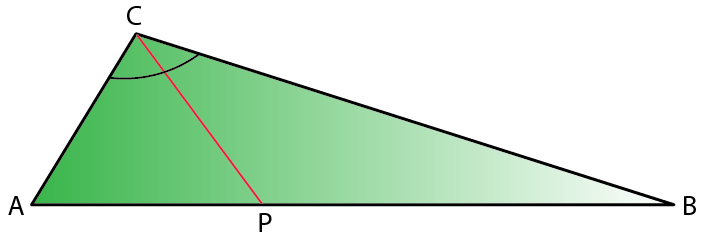Prove: P is equidistant from AC and BC.

Solution: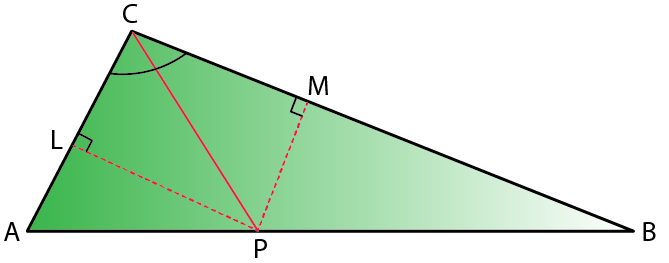Construction: From P, draw PL ⊥ AC and PM ⊥ CB

Proof:

In ∆LPC and ∆MPC,

∠PLC = ∠PMC [Each 90o]

∠PCL = ∠MCP [Given]

PC = PC [Common]

Thus, by AAS criterion of congruence

∆LPC ≅ ∆MPC

And, by Corresponding Parts of Congruent Triangle (CPCT)

PL = PM

Therefore, P is equidistant from AC and BC.

3. Given: AX bisects angle BAC and PQ is perpendicular bisector of AC which meets AX at point Y.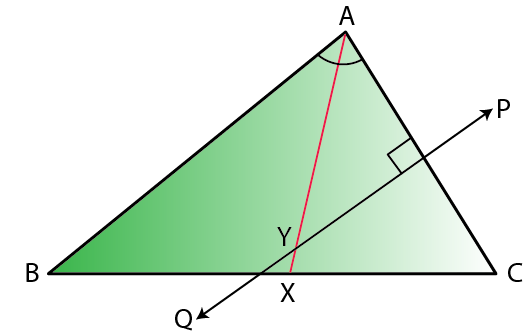Prove:

i) X is equidistant from AB and AC.

ii) Y is equidistant from A and C

Solution: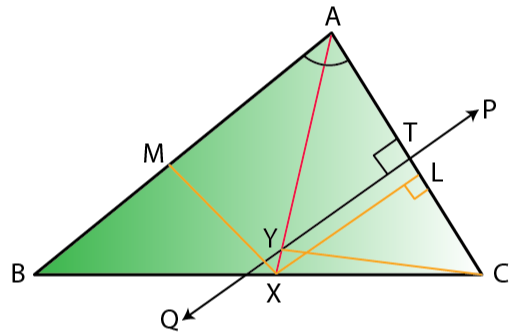Construction: From X, draw XL ⊥ AC and XM ⊥ AB.

And, join YC

Proof:

(i) In ∆AXL and ∆AXM,

∠XAL = ∠XAM [Given]

AX = AX [Common]

∠XLA = ∠XMA [Each 90o]

Thus, by ASA criterion of congruence

∆AXL ≅ ∆AXM

And, by Corresponding Parts of Congruent Triangle (CPCT)

XL = XM

Therefore, X is equidistant from AC and AB.

(ii) In ∆YTA and ∆YTC,

AT = CT [because PQ is perpendicular bisector of AC]

∠YTA = ∠YTC [Each 90o]

YT = YT [Common]

Thus, by SAS criterion of congruence

∆YTA ≅ ∆YTC

And, by Corresponding Parts of Congruent Triangle (CPCT)

YA = YC

Therefore, Y is equidistant from A and C.

4. Construct a triangle ABC, in which AB = 4.2 cm, BC = 6.3 cm and AC = 5 cm. Draw perpendicular bisector of BC which meets AC at point D. Prove that D is equidistant from B and C.

Solution: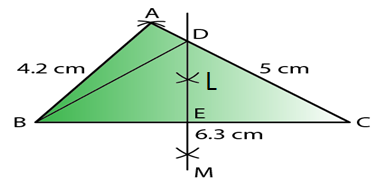Given: In triangle ABC, AB = 4.2 cm, BC = 6.3 cm and AC = 5 cm

Steps of Construction:

i) Draw a line segment BC = 6.3 cm

ii) With centre B and radius 4.2 cm, draw an arc.

iii) With centre C and radius 5 cm, draw another arc which intersects the first arc at A.

iv) Join AB and AC.

Then, ∆ABC is the required triangle.

v) Again with centre B and C and radius greater than ½ BC, draw arcs which intersects each other at L and M.

vi) Join LM intersecting AC at D and BC at E.

vii) Join DB.

Proof:

In ∆DBE and ∆DCE,

BE = EC [LM is the bisector of BC]

∠DEB = ∠DEC [Each 90o]

DE = DE [Common]

Thus, by SAS criterion of congruence

∆DBE ≅ ∆DCE

And, by Corresponding Parts of Congruent Triangle (CPCT)

DB = DC

Therefore, D is equidistant from B and C.

5. In each of the given figures: PA = PB and QA = QB

(i)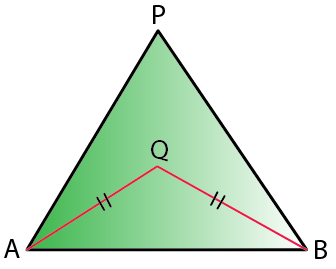(ii)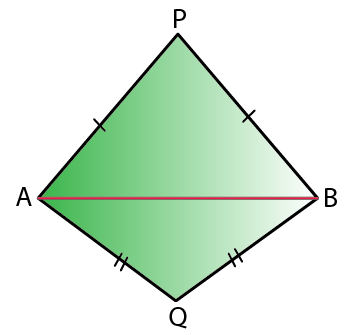Prove in each case, that PQ (produced, if required) is perpendicular bisector of AB.

Hence, state the locus of the points equidistant from two given fixed points.

Solution:

Construction: Join PQ which meets AB in D.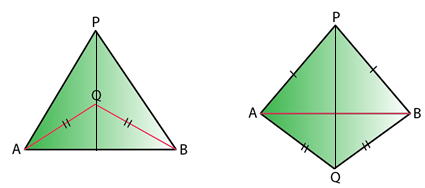Proof:

As P is equidistant from A and B.

Thus, P lies on the perpendicular bisector of AB.

Similarly, as Q is equidistant from A and B.

Q lies on perpendicular bisector of AB.

Thus, both P and Q lie on the perpendicular bisector of AB.

Therefore, PQ is the perpendicular bisector of AB.

Hence, locus of the points which are equidistant from two fixed points, is a perpendicular bisector of the line joining the fixed points.

6. Construct a right angled triangle PQR, in which ∠Q = 90o, hypotenuse PR = 8 cm and QR = 4.5 cm. Draw bisector of angle PQR and let it meet PR at point T. Prove that T is equidistant from PQ and QR.

Solution: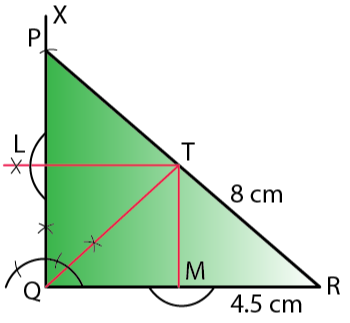Steps of Construction:

i) Draw a line segment QR = 4.5 cm

ii) At Q, draw a ray QX making an angle of 90o

iii) With centre R and radius 8 cm, draw an arc which intersects QX at P.

iv) Join RP.

∆PQR is the required triangle.

v) Draw the bisector of ∠PQR which meets PR at T.

vi) From T, draw perpendicular TL and TM respectively on PQ and QR.

Proof:

In ∆LTQ and ∆MTQ

∠TLQ = ∠TMQ [Each 90o]

∠LQT = ∠TQM [QT is angle bisector]

QT = QT [Common]

Thus, by AAS criterion of congruence

∆LTQ ≅ ∆MTQ

And, by Corresponding Parts of Congruent Triangle (CPCT)

TL = TM

Therefore, T is equidistant from PQ and QR.

7. Construct a triangle ABC in which angle ABC = 75o, AB = 5cm and BC = 6.4 cm. Draw perpendicular bisector of side BC and also the bisector of angle ACB. If these bisectors intersect each other at point P; prove that P is equidistant from B and C; and also from AC and BC.

Solution: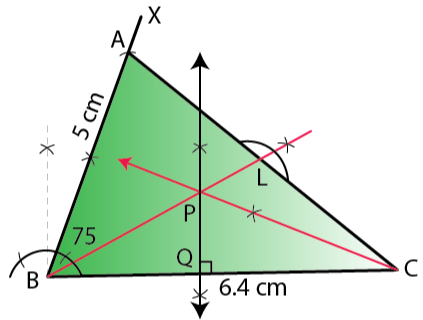Steps of Construction:

i) Draw a line segment BC = 6.4 cm

ii) At B, draw a ray BX making an angle of 75o with BC and cut off BA = 5 cm.

iii) Join AC.

∆ABC is the required triangle.

iv) Draw the perpendicular bisector of BC.

v) Draw the angle bisector of angle ACB which intersects the perpendicular bisector of BC at P.

vi) Join PB and draw PL ⊥ AC.

Proof:

In ∆PBQ and ∆PCQ,

PQ = PQ [Common]

∠PQB = ∠PQC [Each 90o]

BQ = QC [PQ is the perpendicular bisector of BC]

Thus, by SAS criterion of congruence

∆PBQ ≅ ∆PCQ

And, by Corresponding Parts of Congruent Triangle (CPCT)

PB = PC

Therefore, P is equidistant from B and C.

Also,

In ∆PQC and ∆PLC,

∠PQC = ∠PLC [Each 90o]

∠PCQ = ∠PCL [Given]

PC = PC [Common]

Thus, by AAS criterion of congruence

∆PQC ≅ ∆PLC

And, by Corresponding Parts of Congruent Triangle (CPCT)

PQ = PL

Therefore, P is equidistant from AC and BC.

8. In parallelogram ABCD, side AB is greater than side BC and P is a point in AC such that PB bisects angle B. Prove that P is equidistant from AB and BC.

Solution: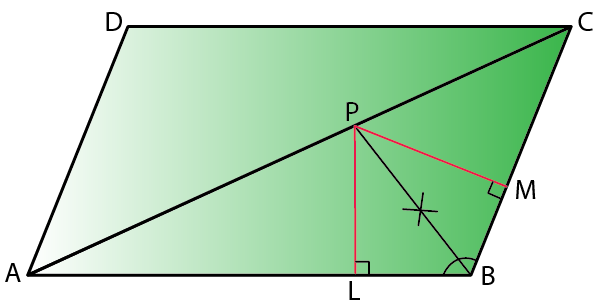Construction: From P, draw PL ⊥ AB and PM ⊥ BC

Proof:

In ∆PLB and ∆PMB,

∠PLB = ∠PMB [Each 90o]

∠PBL = ∠PBM [Given]

PB = PB [Common]

Thus, by AAS criterion of congruence

∆PLB ≅ ∆PMB

And, by Corresponding Parts of Congruent Triangle (CPCT)

PL = PM

Therefore, P is equidistant from AB and BC.

9. In triangle LMN, bisectors of interior angles at L and N intersect each other at point A.

Prove that:

i) point A is equidistant from all the three sides of the triangle.

ii) AM bisects angle LMN.

Solution: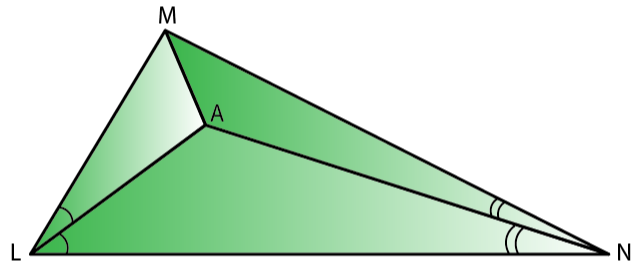Construction: Join AM

Proof:

(i) Since, A lies on bisector of ∠N

So, A is equidistant from MN and LN.

Again, as A lies on the bisector of ∠L

A is equidistant from LN and LM.

Therefore, A is equidistant from all three sides of the triangle LMN.

And,

(ii) As A lies on the bisector of ∠M.

Hence, AM bisects ∠LMN

10. Use ruler and compasses only for this question.

(i) Construct ∆ABC, where AB = 3.5 cm, BC = 6 cm and ∠ABC = 60o

(ii) Construct the locus of points inside the triangle which are equidistant from BA and BC.

(iii) Construct the locus of points inside the triangle which are equidistant from B and C.

(iv) Mark the point P which is equidistant from AB, BC and also equidistant from B and C. Measure and record the length of PB.

Solution: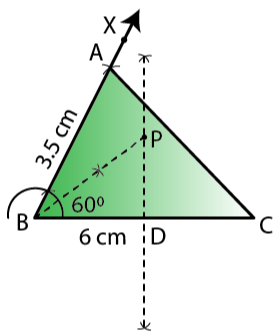Steps of construction:

(i) Draw line BC = 6 cm and construct angle CBX = 60o. Cut off AB = 3.5. Join AC, triangle ABC is the required triangle.

(ii) Draw the perpendicular bisector of BC and bisector of angle B.

(iii) Now, bisector of angle B meets bisector of BC at P.

Hence, BP is the required length, where PB = 3.5 cm.

(iv) P is the point which is equidistant from BA and BC, which is also equidistant from B and C.

PB = 3.5 cm

### Exercise 16(B) Page No: 240

Describe the locus for questions 1 to 13 given below:

1. The locus of a point at a distance of 3 cm from a fixed point.

Solution: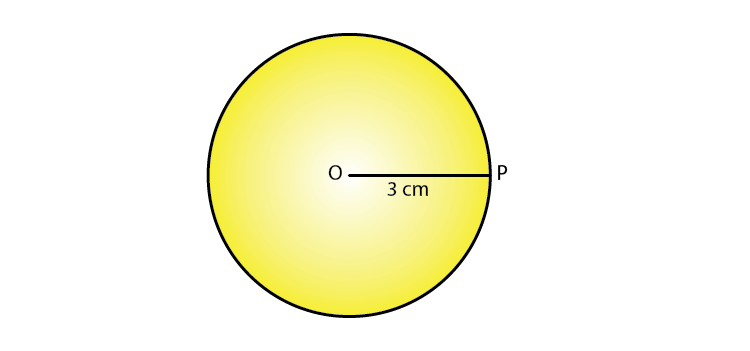The locus of a point which is at a distance of 3 cm away from a fixed point is circumference of a circle whose radius is 3 cm and the fixed point is the centre of the circle.

2. The locus of a points at a distance of 2 cm from a fixed line.

Solution: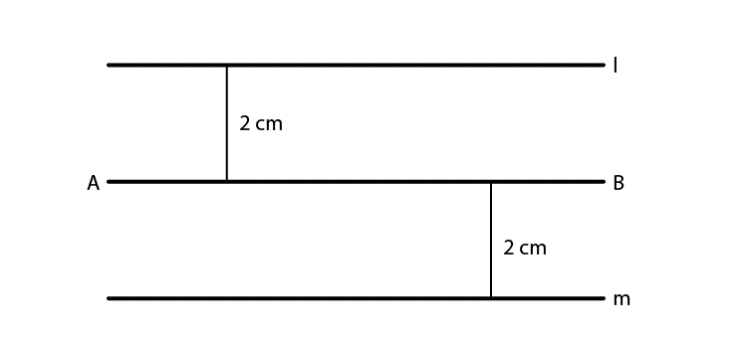The locus of points which are at a distance of 2 cm from a fixed line AB are a pair of straight lines l and m which are parallel to the given line at a distance of 2 cm.

3. The locus of the centre of a wheel of a bicycle going straight along a level road.

Solution: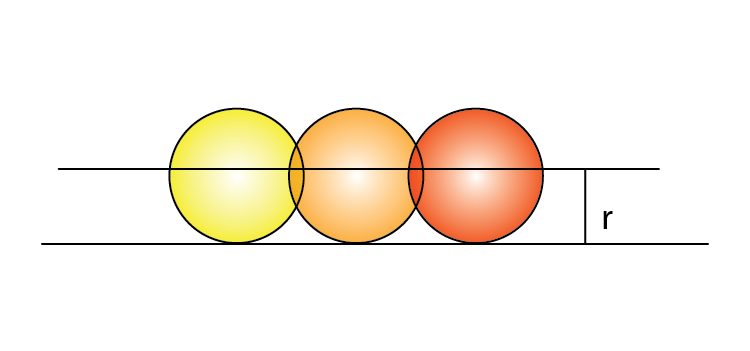The locus of the centre of a wheel, which is going straight along a level road will be a straight line parallel to the road at a distance equal to the radius of the wheel.

4. The locus of the moving end of the minute hand of a clock.

Solution: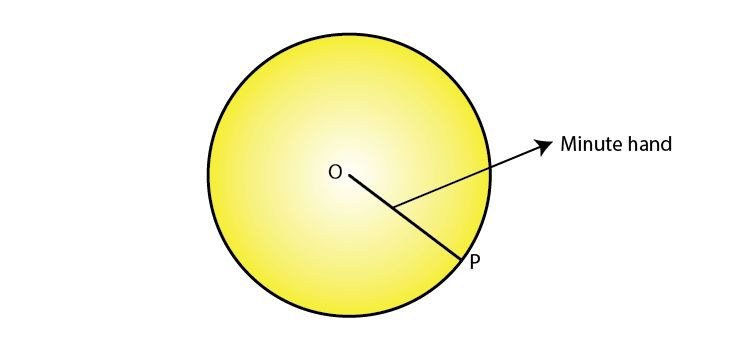The locus of the moving end of the minute hand of the clock will be a circle whose radius will be the length of the minute hand.

5. The locus of a stone dropped from the top of a tower.

Solution: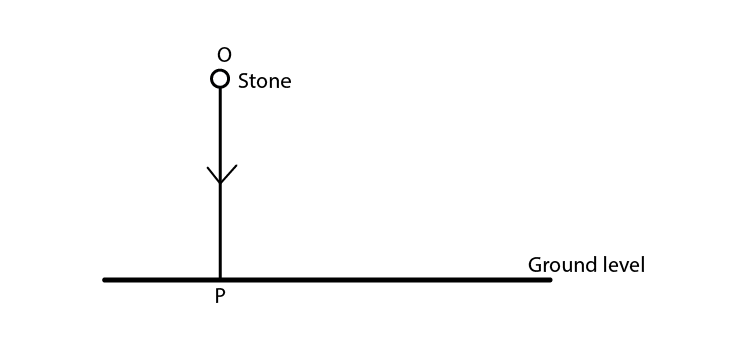The locus of a stone which is dropped from the top of a tower will be a vertical line through the point from which the stone is dropped.

6. The locus of a runner, running around a circular track and always keeping a distance of 1.5 m from the inner edge.

Solution: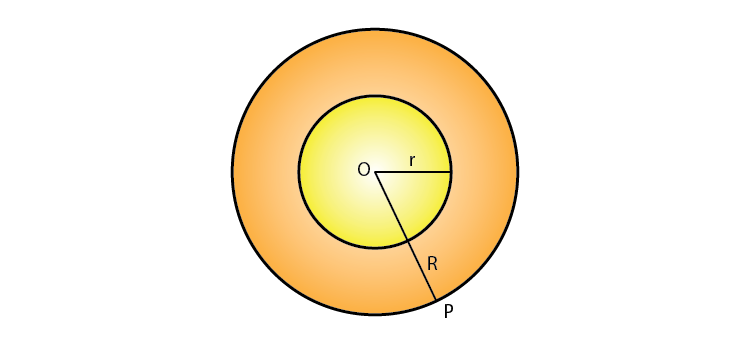The locus of the runner, running around a circular track and always keeping a distance of 1.5 m from the inner edge will be the circumference of a circle where the radius is equal to the radius of the inner circular track and exceeding 1.5 m.

7. The locus of the door-handle, as the door opens.

Solution: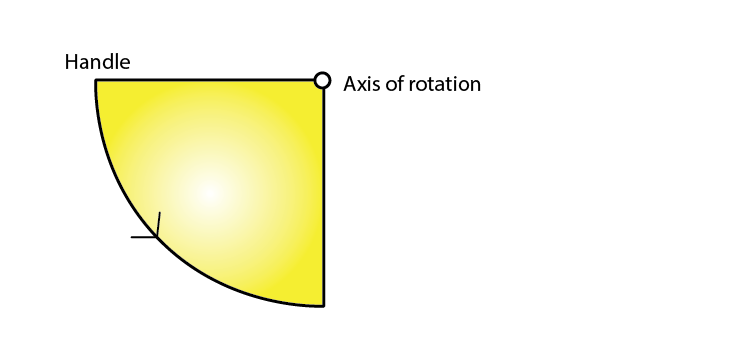The locus of the door handle will be the circumference of a circle with centre at the axis of rotation of the door and the radius equal to distance between the door handle and the axis of rotation of the door.

8. The locus of a points inside a circle and equidistant from two fixed points on the circumference of the circle.

Solution: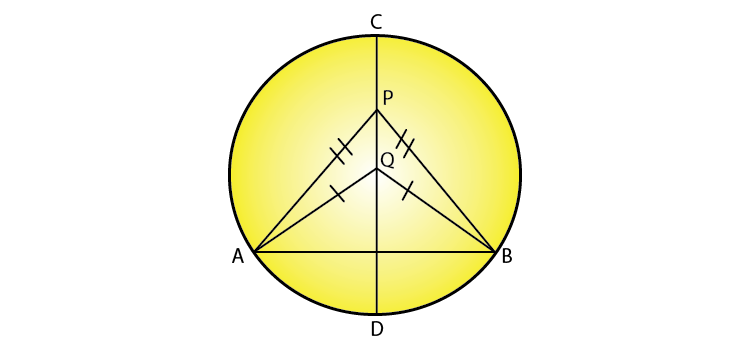The locus of the points inside the circle which are equidistant from the fixed points on the circumference of a circle will be a diameter which is the perpendicular bisector of the line joining the two fixed points on the circle.

9. The locus of the centres of all circles passing through two fixed points.

Solution: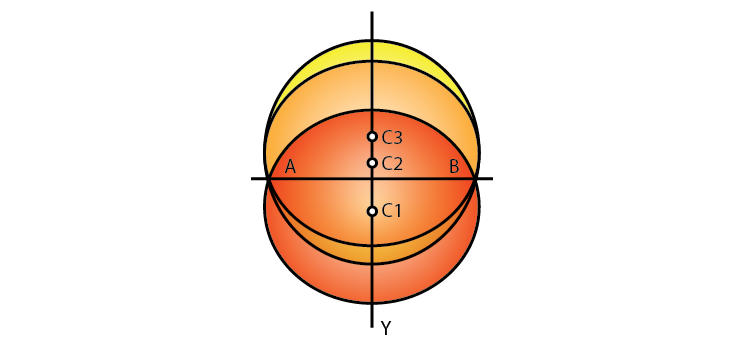The locus of the centres of all the circles passing through two fixed points will be the perpendicular bisector of the line segment joining the two given fixed points.

10. The locus of vertices of all isosceles triangles having a common base.

Solution: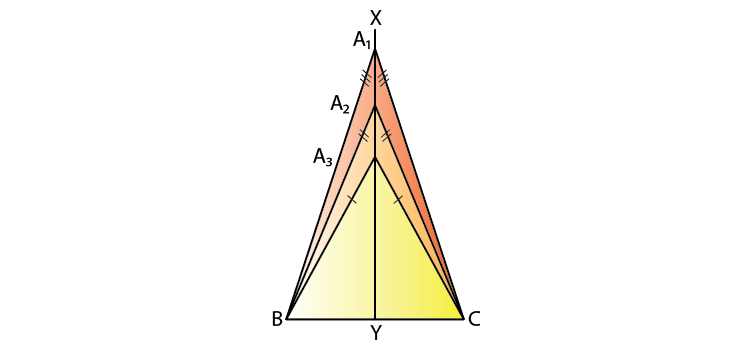The locus of vertices of all isosceles triangles having a common base will be the perpendicular bisector of the common base of the triangles.

11. The locus of a point in space which is always at a distance of 4 cm from a fixed point.

Solution:

The locus of a point in space is the surface of the sphere whose centre is the fixed point and radius equal to 4 cm.

12. The locus of a point P, so that:

AB2 = AP2 + BP2,

where A and B are two fixed points.

Solution: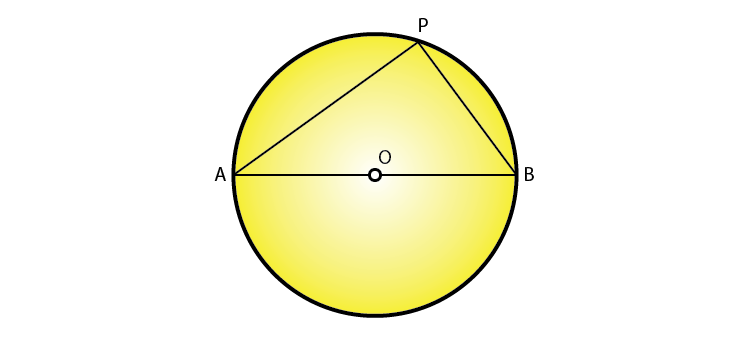The locus of the point P is the circumference of a circle with AB as diameter and satisfies the condition AB2 = AP2 + BP2.

13. The locus of a point in rhombus ABCD, so that it is equidistant from

i) AB and BC; ii) B and D.

Solution:

i)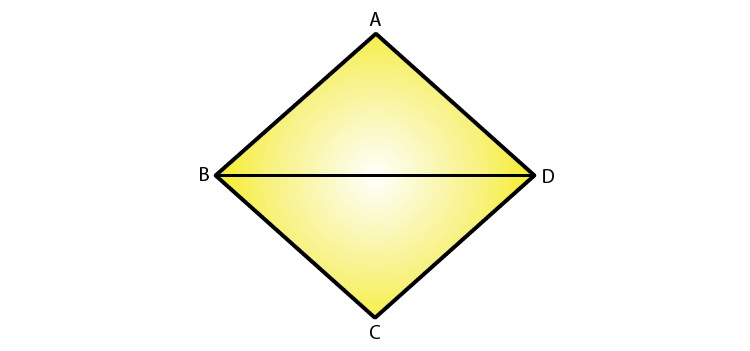The locus of the point in a rhombus ABCD which is equidistant from AB and BC will be the diagonal BD of the rhombus.

ii)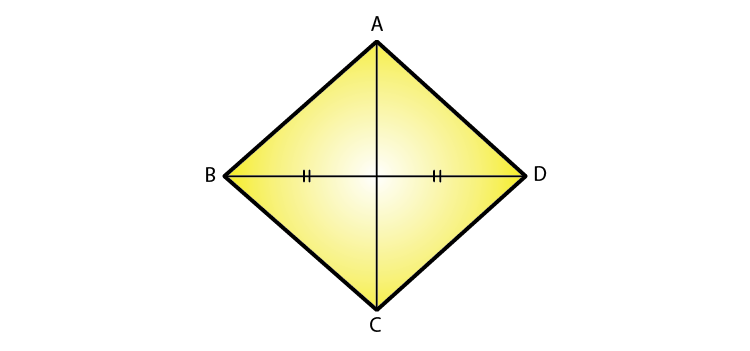The locus of the point in a rhombus ABCD which is equidistant from B and D will be the diagonal AC.

14. The speed of sound is 332 meters per second. A gun is fired. Describe the locus of all the people on the Earth’s surface, who hear the sound exactly one second later.

Solution:

The locus of all the people on Earth’s surface is the circumference of a circle whose radius is 332 m and centre is the point where the gun is fired.

15. Describe:

i) The locus of points at distances less than 3 cm from a given point.

ii) The locus of points at distances greater than 4 cm from a given point.

iii) The locus of points at distances less than or equal to 2.5 cm from a given point.

iv) The locus of points at distances greater than or equal to 35 mm from a given point.

v) The locus of the centre of a given circle which rolls around the outside of a second circle and is always touching it.

vi) The locus of the centres of all circles that are tangent to both the arms of a given angle.

vii) The locus of the mid-points of all chords parallel to a given chord of a circle.

viii) The locus of points within a circle that are equidistant from the end points of a given chord.

Solution:

i) The locus of the points will be the space inside of the circle whose radius is 3 cm and centre as the given point.

ii) The locus of the points will be the space outside of the circle whose radius is 4 cm and centre is the given fixed point.

iii) The locus of points is the space inside and the circumference of the circle with a radius of 2.5 cm and the centre as the given fixed point.

iv) The locus is the space outside and circumference of the circle with a radius of 35 mm and the centre as the given fixed point.

v) The locus is the circumference of the circle concentric with the second circle whose radius is equal to the sum of the radii of the given two circles.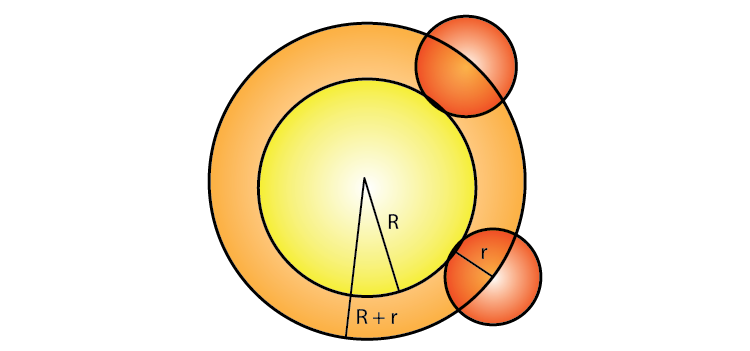vi) The locus of the centre of all circles whose tangents are the arms of a given angle is the bisector of that angle.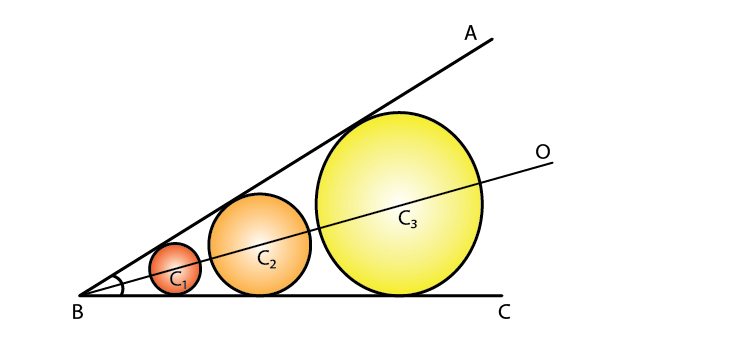vii) The locus of the mid-points of the chords which are parallel to a given chords is the diameter perpendicular to the given chords.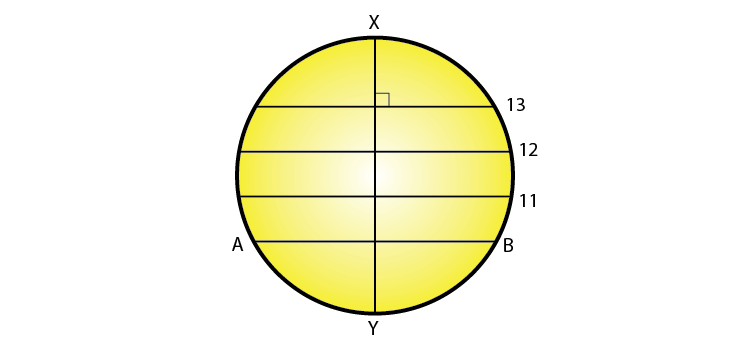viii) The locus of the points within a circle which are equidistant from the end points of a given chord is the diameter which is the perpendicular bisector to the given chord.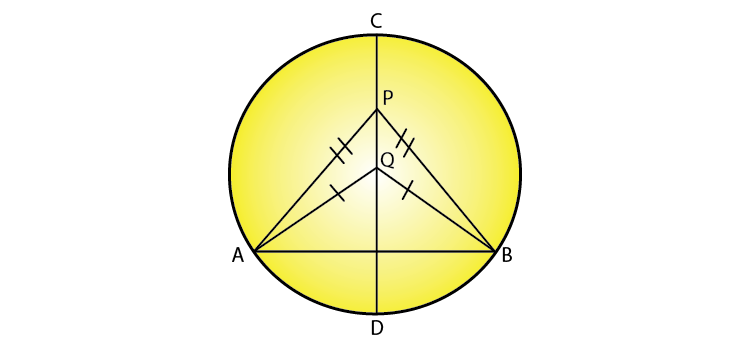### Exercises of Selina Solutions Concise Maths Class 10 Chapter 16 Loci (Locus and its Constructions)

Exercise 16(A) Solutions

Exercise 16(B) Solutions